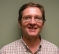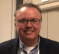## Difference between averaging and decimation on oversampled dataStarted by 7 months ago4 replieslatest reply 7 months ago260 views

In my project I am oversampling the data (12 bit ADC) for the following reasons,

1. To relax the antialiasing filter requirements before the ADC.

2. Increase or decrease the total sampling time to fill fixed buffer (ex. 1024 point array)

To reduce the sampling frequency from the oversampled frequency and to reduce the number of collected samples, i am planning to employ averaging or decimation filter. I am not looking to improve the resolution.

What is the difference between the averaging and using decimation filter?

Ex. Maximum frequency of interest = 2kHz with Nyquist limit of 5kHz, Sampling frequency is set at 10kHz, with a oversampling factor of 2.

Case 1: Averaging - I will collect 1024 data points and average 2 samples to arrive at 512 data points. Now averaging will reduce the sampling rate and number of samples by factor 2. I also believe it will act as a low pass filter. My new sampling frequency is 5kHz.

old data 1 + old data 2 = New data 1

.. .. .. ..

.. .. .. ..

old data 1023 + old data 1024 = New data 512

Case 2: Filtering and Decimation by factor of 2.

I pass the data samples through a FIR filter, to respect the Nyquist criteria for the new sampling frequency(5 kHz) and then decimate by a factor of 2.

old data 1 = new data 1

old data 3 = new data 2

.. .. .. ..

old data 1024 = New data 512

What is the difference between the two approaches described above? I could see averaging reduced the amplitude of the wave to a small extent but that should be ok i believe.  I am not looking to improve the ADC resolution.

[ - ]Often decimation filtering is done very simply with averaging: a CIC decimation filter is mathematically equivalent to a moving average over D samples followed by downsampling (selecting every Dth sample). The scaling in a true average (divide by D) may or may not be done with no consequence other than getting to the desired word width and the truncation noise associated with that. Such a single moving average is a very poor filter (Sinc in frequency) so to get the desired rejection of the alias frequency band regions we must often cascade multiple CIC sections, meaning multiple moving averages in cascade. The result of the cascaded Sinc shaped responses is “passband droop” but this can easily be compensated for with a simple inverse Sinc filter.

In general a decimation filter is required for anti-aliasing; this is no different from anti-aliasing for an ADC. Decimation is just a “digital to digital converter” with the same aliasing mechanism as an ADC. The ideal decimation filter would pass the passband without any distortion and completely reject all the image frequency locations. Ideal is not realizable, but can be arbitrarily approached with filter complexity.

Given the requirement of not being concerned with increasing precision (meaning due to the oversampling there will likely be excess dynamic range), I recommend looking into implementing a CIC decimator given its simplicity, and if the passband droop is an option this can likely be corrected for with a simple 3 tap FIR filter.

I show these concepts in more detail at the following links:

(Side note for the purists: I say "Sinc in frequency" above loosely, the frequency response of a discrete time moving average is the Dirichlet Kernel, which is effectively an aliased Sinc function).

[ - ]Thanks for helping out.

Sorry if i am  being novice, as i am new to digital signal processing domain.

So, if i understand correctly a simple moving average followed by decimation(Select Dth sample and Discard D-1 samples) would not be efficient in removing aliasing to respect the new Nyquist limit and there by introduce folding in the usable frequency region.

And finaly, How this CIC decimator is different from FIR decimator? Since microcontroller CMSIS DSP library provides functions readily for FIR, can i use FIR decimator in place of CIC decimator?

[ - ]Depends on the decimation ratio you need.  For large decimation ratio a CIC is hard to beat for efficiency.  Depending on how flat the frequency response needs to be on the output a final FIR filter can be used to compensate for the CIC droop.

Another option that does use FIRs are canonical half-band filters.

Again, just depends on your application.

Mark Napier

[ - ]Question: What is the difference between the two approaches described above?

The filter is the only difference.  Consider the cases as you describe:

Case 1: Averaging - "I will collect 1024... old data 1 + old data 2 = New data 1"

So you are implementing a two-tap averager on every sample and - then - throwing away every other averaged data point.  Each output data value equals the average of the past two.  A two-tap averager is an FIR filter that has a transfer function of y(z)/x(z)=(1+z^-1)/2 and has the following frequency response:As you surmised, a two-tap averager is a (somewhat lame) lowpass filter.  The fact that you are calculating the output for each timestep is somewhat immaterial/wasteful because you are discarding every other output (i.e., you are decimating).

Case 2: Filtering and Decimation by factor of 2 - "I pass the data samples through a FIR filter, to respect the Nyquist criteria for the new sampling frequency(5 kHz) and then decimate by a factor of 2."

That's what you did in Case 1.  The only difference is that - presumably - in case 2 you will use a more capable lowpass filter.

So... which "case" you choose to do is simply a matter of what pre-decimation filter response you desire.  Case 1 is also wasteful in that 1/2 of the filter computations are unnecessary.  ...at least as described.

But, in both cases, you have an FIR filter with a 2x decimation chaser.  That's my interpretation of what you wrote, anyway.